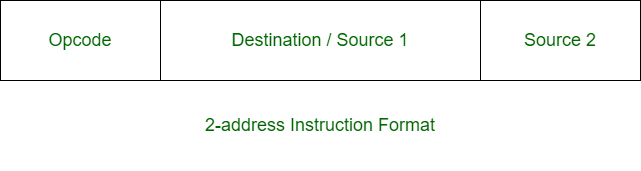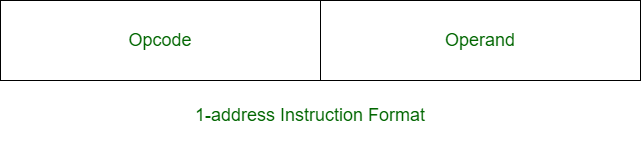Open in App
Not now

• Last Updated : 19 Jun, 2020

Prerequisite – Instruction Formats
Two-address instruction is a format of machine instruction. It has one opcode and two address fields. One address field is common and can be used for either destination or source and other address field for source.Example:

`X = (A + B) x (C + D) `

Solution:

```MOV R1, A      R1 <- M[A]
ADD R1, B      R1 <- R1 + M[B]
MOV R2, C      R2 <- M[C]
ADD R2, D      R2 <- R2 + D
MUL R1, R2     R1 <- R1 x R2
MOV X, R1      M[X] <- R1 ```

One-Address instruction is also a format of machine instruction. It has only two fields. One for opcode and other for operand.Example:

`X = (A + B) x (C + D) `

Solution:

```LOAD A      AC <- M[A]
ADD B       AC <- AC + M[B]
STORE T     M[T] <- AC
ADD D       AC <- AC + M[D]
MUL T       AC <- AC x M[T]
STORE X     M[X] <- AC  ```